Mastering the Minor Pentatonic Scale – Video Lesson

The Minor Pentatonic Scale

The minor pentatonic scale is often one of the first scales learned by guitar players, and certainly one that is very frequently used. It's provided the melodic foundation to some of the most classic solos and riffs of all time, and is found in almost all styles of music. In order to master this scale, you must first understand how it's constructed and how each note in the scale functions. You should then familiarize yourself with each position on the fretboard and be able to maneuver between shapes.

More...

Construction

The minor pentatonic scale is a five-note scale ("penta" meaning five) that can be built by using the following formula: 1-b3-4-5-b7. If you are new to scale and chord construction, you may be asking yourself what to do with a bunch of numbers. In order to apply a formula, you must have something to apply it to. In Western harmony & theory, the answer is the Major Scale, as it serves as the foundation of every scale and chord you will learn. In this case, we are going to build an A minor pentatonic scale; therefore, we will begin with the notes from the A major scale.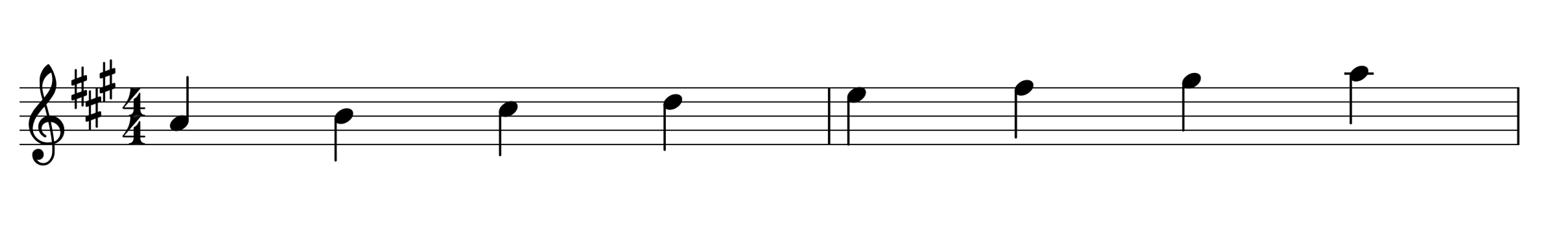The key of A major has 3 sharps, giving us the follow notes: A-B-C#-D-E-F#-G#. Each scale degree is then given a number (interval). In this case, A is 1, B is 2, C# is 3, D is 4, E is 5, F# is 6, and G# is 7 (we can label the A an octave higher than our root as 8 to complete the octave). So, now that we have numbers 1 though 8 and notes that correspond with each of those numbers, we can apply our formula to construct an A minor pentatonic scale.

Applying the formula, we begin with 1, which in this case is an A note. Now we have a b3 (pronounced "flat 3"), but there is no b3 in the major scale. The word "flat" instructs us to lower a note by a half-step (or one fret). If we lower the 3rd of A major (C#) one half step, we get the note C. Moving along in our formula, we get a 4 which is in our A major scale and is the note D. Next, is a fifth (or 5), and in the key of A major is an E note. Our last interval in our formula calls for a b7 (pronounced "flat 7" or minor seventh). Again, since we don't have a b7 in our A major scale, we are going to have to lower the 7th (G#) by one half step, which gives us a G note. Our formula is now complete, and we are left with the follow notes: A-C-D-E-G, which make up an A minor pentatonic scale.

Another way of thinking of the minor pentatonic scale, is to begin with a natural minor scale (the formula for a natural minor scale is 1-2-b3-4-5-b6-b7) and remove the 2nd and b6th notes, which leaves us with the following formula: 1-b3-4-5-b7. It's important to know how to construct scales and chords from scratch, but once you start learning more scales and chords, it's useful to see how things connect and fit together.

The Five CAGED Positions

Now that we know how to construct a minor pentatonic scale, let's see the various ways we can play it on the guitar. There are five shapes of the pentatonic scale, each one relating to one of the five open chord shapes (the C shape, A shape, G shape, E shape, and D shape). By laying these five positions across the fretboard, we are able to cover the entire fretboard. Let's take a look at each shape.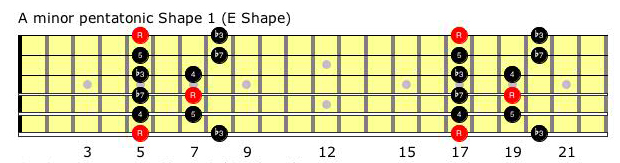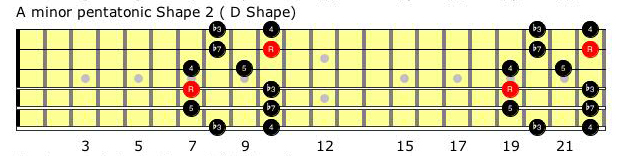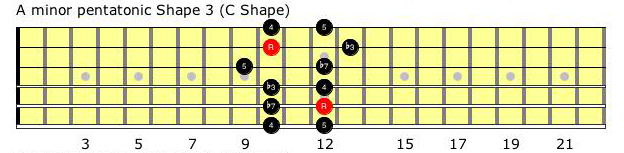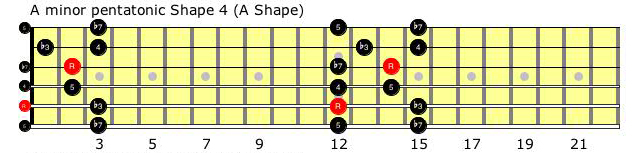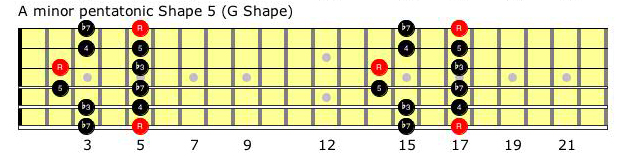Once you've become familiar with each shape individually, see if you can combine the shapes laterally (vertically) on the fretboard. The minor pentatonic scale is used all the time in music, especially in Blues, Rock, and Fusion. Not only will mastering the minor pentatonic scale improve your guitar playing, it is sure to improve your knowledge of the fretboard. Work on nailing these 5 shapes, and most importantly, have fun!